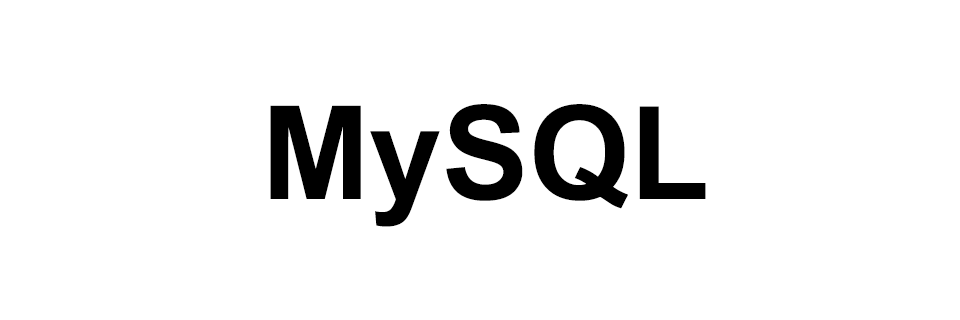# MySQL - Data Types

MySQLIn this tutorial we will learn about some of the commonly used data types in MySQL.

Data types of a column tells us about the type of data stored in the column.

In this tutorial we will be discussing the following three types.

## Numeric Types

Data types under this category holds numerical data.

### Integer Data Types

They hold exact integer values like 10, 0, -100 etc. and we can summaries the range and size of the data type as follows.

We can also use `UNSIGNED` to store unsigned value like 0, 10, 20, 100 etc. No negative value.

Data TypeSize (byte)Min value (signed)Max value (signed)Min value (unsigned)Max value (unsigned)
TINYINT1-1281270255
SMALLINT2-3276832767065535
MEDIUMINT3-83886088388607016777215
INT4-2147483648214748364704294967295
BIGINT8-92233720368547758089223372036854775807018446744073709551615

## Floating-Point Data Types

We use this data type to store approximate value with decimal part.

For single precision values we use `FLOAT` which takes 4 bytes space.

For double precision values we use `DOUBLE` which takes 8 bytes space.

Syntax: `FLOAT(M,N)`

Here M represents the total number of digits that can be stored. Whereas, N represents the total number of digits after the decimal point.

Example: `velocity FLOAT(10,4)`

This means we can store total 10 digits out of which 4 digits can be stored after the decimal point. Like: -10.1234 or 999.4321 etc.

## String Types

Data types in this category are used to hold string value.

### Char and Varchar Data Types

`CHAR` and `VARCHAR` data types are used to store string values.

### CHAR

`CHAR` data type gives us a fixed width column size.

Syntax: `CHAR(N)`

Where, N is the total number of characters we can save in the column. Range of N = 0 to 255.

Size is also equal to N so, CHAR(10) means it can hold 10 characters and column size is 10 bytes.

Note! `CHAR(4)` can hold max 4 characters. But if less characters are entered then it is right padded with white space and saved in the column. And when the value is retrieved the trailing right white spaces are removed.

### VARCHAR

`VARCHAR` data type gives us a variable width column size.

Syntax: `VARCHAR(N)`

Where, N is the total number of characters we can save in the column. Range of N = 0 to 65535.

If N <= 255 then, size of the column VARCHAR(N) is 1+N bytes. So, value is saved as 1 byte prefix plus data bytes.

And if N > 255 then, size of the column VARCHAR(N) is 2+N bytes. So, value is saved as 2 bytes prefix plus data bytes.

So, the prefix byte tells about the length of the data saved in the VARCHAR column.

Trailing spaces in case of VARCHAR is retained when saved in the column and when retrived from the column.

Example: VARCHAR(100) if assigned a string value "Hello" will take up 6 bytes, 5 for the string "Hello" and 1 for the prefix byte.

### Enum Data Types

`ENUM` is a string object that can take value from a list of permitted values.

Syntax: `ENUM('value1', 'value2', ...)`

Max number of values: 65535.

Example: A user account can be in ACTIVE, INACTIVE and DELETED state and we can denote this using ENUM as follows `account_status ENUM('ACTIVE', 'INACTIVE', 'DELETED');`.

### Blob and Text Data Types

`BLOB` is a binary large object and is used to save data as byte (binary) string. There are 4 types of BLOB variation namely `TINYBLOB`, `BLOB`, `MEDIUMBLOB` and `LONGBLOB`.

`TEXT` is used to save data as character (non-binary) string. There are 4 types of TEXT variation namely `TINYTEXT`, `TEXT`, `MEDIUMTEXT` and `LONGTEXT`.

TypeStorage size
TINYBLOB, TINYTEXTL + 1 bytes, where L < 28
BLOB, TEXTL + 2 bytes, where L < 216
MEDIUMBLOB, MEDIUMTEXTL + 3 bytes, where L < 224
LONGBLOB, LONGTEXTL + 4 bytes, where L < 232

Where, L represents the actual length (in bytes) of a given string value.

Example: If a column data type is BLOB then its size is L+3. THe extra 3 bytes is to store the length of the value and L is the actual length (in bytes) of a given string value.

## Date and Time type

Data types of this category is used to store date and time value.

### Date Data Types

We use the `DATE` data type when we want to store date value.

Syntax: 'YYYY-MM-DD' where, YYYY is year 1000-9999, MM is month 1-12 and DD is date 1-31.

So, the range for `DATE` is '1000-01-01' to '9999-12-31'.

### Datetime Data Types

We use the `DATETIME` data type when we want to store date and time value.

Syntax: 'YYYY-MM-DD HH-MM-SS' where, YYYY is year 1000-9999, MM is month 1-12 and DD is date 1-31.

HH is hour 00-23, MM is minute 00-59 and SS is second 00-59.

So, the range for `DATETIME` is '1000-01-01 00:00:00' to '9999-12-31 23:59:59'.

### Timestamp Data Types

We use the `TIMESTAMP` data type to store value that have date and time part.

Syntax: 'YYYY-MM-DD HH-MM-SS' where, YYYY is year 1000-9999, MM is month 1-12 and DD is date 1-31.

HH is hour 00-23, MM is minute 00-59 and SS is second 00-59.

So, the range for `TIMESTAMP` is '1970-01-01 00:00:01' UTC to '2038-01-19 03:14:07' UTC.